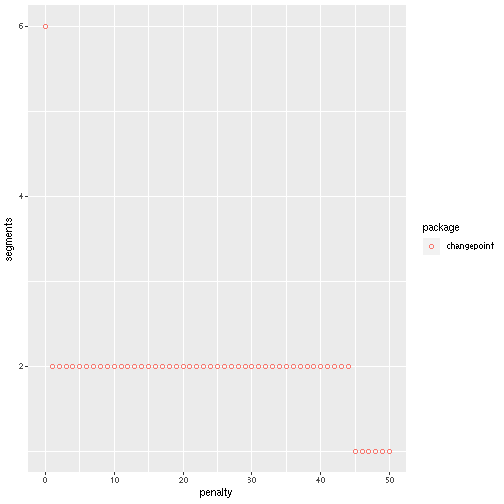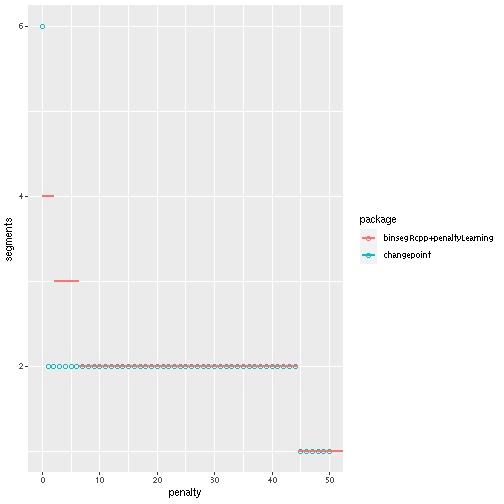# Comparisons

## Comparing loss/parameters to changepoint

The code below shows how to run the binary segmentation algorithm for the model with a change in normal mean and variance.

``````x <- c(0,0.3,0.2,0.1, 10,11,12,13)
(bs.fit <- binsegRcpp::binseg("meanvar_norm", x))
#> binary segmentation model:
#>    segments end       loss validation.loss
#> 1:        1   8 25.3177168               0
#> 2:        2   4  3.0337421               0
#> 3:        3   6 -0.1851337               0
#> 4:        4   2 -1.2067850               0
``````

The code below computes the theoretical expected loss, which is the same as computed by binsegRcpp:

``````myvar <- function(y)mean((y-mean(y))^2)
nll <- function(y)-sum(dnorm(y, mean(y), sqrt(myvar(y)), log=TRUE))
expected.loss <- c(
nll(x),
nll(x[1:4])+nll(x[5:8]),
nll(x[1:4])+nll(x[5:6])+nll(x[7:8]),
nll(x[1:2])+nll(x[3:4])+nll(x[5:6])+nll(x[7:8]))
rbind(binsegRcpp=bs.fit\$splits\$loss, expected=expected.loss)
#>                [,1]     [,2]       [,3]      [,4]
#> binsegRcpp 25.31772 3.033742 -0.1851337 -1.206785
#> expected   25.31772 3.033742 -0.1851337 -1.206785
``````

The code below runs binary segmentation from the changepoint package for comparison:

``````cpt.fit <- changepoint::cpt.meanvar(
x, penalty="Manual", pen.value=0, method="BinSeg")
#> Warning in BINSEG(sumstat, pen = pen.value, cost_func = costfunc, minseglen
#> = minseglen, : The number of changepoints identified is Q, it is advised to
#> increase Q to make sure changepoints have not been missed.
changepoint::logLik(cpt.fit)
#> Warning in .local(object, ...): Not changed to be -2*logLik
#>    -like -likepen
#>      NaN      NaN
``````

The code above shows that the changepoint package logLik method returns NaN rather than a finite value.

``````changepoint::param.est(cpt.fit)
#> \$mean
#>   0.00  0.00  0.00  0.00  0.15 11.50
#>
#> \$variance
#>      NA     NA     NA     NA 0.0125 1.2500
coef(bs.fit, 2L)
#>    segments start end start.pos end.pos  mean    var
#> 1:        2     1   4       0.5     4.5  0.15 0.0125
#> 2:        2     5   8       4.5     8.5 11.50 1.2500
``````

The code above shows that changepoint and binsegRcpp mean/variance estimates (for 2 segments) are consistent, but changepoint result contains extra 0/NA values.

``````cpt.fit1 <- changepoint::cpt.meanvar(
x, penalty="Manual", pen.value=0, method="BinSeg", Q=1)
#> Warning in BINSEG(sumstat, pen = pen.value, cost_func = costfunc, minseglen
#> = minseglen, : The number of changepoints identified is Q, it is advised to
#> increase Q to make sure changepoints have not been missed.
changepoint::param.est(cpt.fit1)
#> \$mean
#>   0.15 11.50
#>
#> \$variance
#>  0.0125 1.2500
``````

The code above shows that changepoint package binary segmentation returns reasonable parameter estimates if Q=1 changepoint is specified.

``````rbind(
changepoint=changepoint::logLik(cpt.fit1)/2,
binsegRcpp=bs.fit\$splits\$loss)
#> Warning in .local(object, ...): Not changed to be -2*logLik
#>                -like -likepen
#> changepoint 3.033742 3.033742
#> binsegRcpp  3.033742 3.033742
``````

The code above shows that the changepoint loss (negative log likelihood) differs from binsegRcpp/dnorm by a factor of 2.

Note below that binsegRcpp also can compute parameter estimates for other model sizes (not limited to 2 segments in this data set),

``````coef(bs.fit)
#>     segments start end start.pos end.pos   mean      var
#>  1:        1     1   8       0.5     8.5  5.825 32.83687
#>  2:        2     1   4       0.5     4.5  0.150  0.01250
#>  3:        2     5   8       4.5     8.5 11.500  1.25000
#>  4:        3     1   4       0.5     4.5  0.150  0.01250
#>  5:        3     5   6       4.5     6.5 10.500  0.25000
#>  6:        3     7   8       6.5     8.5 12.500  0.25000
#>  7:        4     1   2       0.5     2.5  0.150  0.02250
#>  8:        4     3   4       2.5     4.5  0.150  0.00250
#>  9:        4     5   6       4.5     6.5 10.500  0.25000
#> 10:        4     7   8       6.5     8.5 12.500  0.25000
``````

# Penalized model selection with binsegRcpp and changepoint

Sometimes we don't know the desired number of changepoints/segments, but we can specify a non-negative penalty value, and we want to select the number of changes which minimizes the total cost (loss + penalty * number of changes). In that case we can do the following:

``````penaltyLearning::modelSelection(bs.fit\$splits, "loss", "segments")
#> Warning: replacing previous import 'lifecycle::last_warnings' by
#> Warning: replacing previous import 'lifecycle::last_warnings' by
#>   min.lambda max.lambda min.log.lambda max.log.lambda cum.iterations segments
#> 4   0.000000   1.021651           -Inf     0.02142019              3        4
#> 3   1.021651   3.218876     0.02142019     1.16903218              2        3
#> 2   3.218876  22.283975     1.16903218     3.10386779              1        2
#> 1  22.283975        Inf     3.10386779            Inf              0        1
#>         loss validation.loss end depth before.mean before.var after.mean
#> 4 -1.2067850               0   2     2       0.150    0.02250       0.15
#> 3 -0.1851337               0   6     2      10.500    0.25000      12.50
#> 2  3.0337421               0   4     1       0.150    0.01250      11.50
#> 1 25.3177168               0   8     0       5.825   32.83687         NA
#>   after.var before.size after.size invalidates.index invalidates.after
#> 4    0.0025           2          2                 2                 0
#> 3    0.2500           2          2                 2                 1
#> 2    1.2500           4          4                 1                 0
#> 1        NA           8         NA                NA                NA
``````

The code above computes a model selection data frame, where every row is a model size, and the min.lambda/max.lambda columns indicate the penalty values which select that model size. For example if you wanted to use a penalty value of 5 then that select the model with 2 segments, since 5 is between min.lambda=3.21 and max.lambda=22.28.

How could we do something similar with changepoint package? We could try the CROPS penalty, but that only works with the PELT method (not binary segmentation),

``````try(changepoint::cpt.meanvar(
x, penalty="CROPS", method="BinSeg", pen.value = c(0, Inf)))
#> Error in CROPS(data = data, method = method, pen.value = pen.value, test.stat = test.stat,  :
#>   CROPS is a valid penalty choice only if method="PELT", please change your method or your penalty.
``````

Instead we could write a for loop over potential penalty values,

``````pen.changepoint.list <- list()
for(penalty in seq(0, 50)){
pen.fit <- changepoint::cpt.meanvar(
x, penalty="Manual", method="BinSeg", pen.value=penalty)
pen.changepoint.list[[paste(penalty)]] <- data.frame(
package="changepoint",
segments=length(changepoint::cpts(pen.fit))+1L,
penalty)
}
#> Warning in BINSEG(sumstat, pen = pen.value, cost_func = costfunc, minseglen
#> = minseglen, : The number of changepoints identified is Q, it is advised to
#> increase Q to make sure changepoints have not been missed.
pen.changepoint <- do.call(rbind, pen.changepoint.list)
library(ggplot2)
(gg.penalty <- ggplot()+
geom_point(aes(
penalty, segments, color=package),
shape=1,
data=pen.changepoint))
``````The figure above shows that the changepoint package returns 1 segment for large penalty values, 2 segments for intermediate penalty values, and 6 segments (with 0/NA values) when penalty=0. The method above involves running the binary segmentation algorithm for each penalty value, which is not necessary. It is more efficient to run the binary segmentation algorithm once, and then analyze the loss values to determine which penalty values select which model sizes, as is done below with binsegRcpp and penaltyLearning. We re-run the modelSelection function below for direct comparison with the changepoint results (changepoint loss/penalty values are off by a factor of two),

``````library(data.table)
models <- data.table(
package="binsegRcpp+penaltyLearning",
bs.fit\$splits
)[, cpt.loss := loss*2]
pen.df <- penaltyLearning::modelSelection(models, "cpt.loss", "segments")
gg.penalty+
geom_segment(aes(
min.lambda, segments,
color=package,
xend=max.lambda, yend=segments),
size=1,
data=pen.df)
``````The figure above shows that binsegRcpp+modelSelection and changepoint packages are consistent for penalties larger than about 10. However for some small penalty values, between 0 and 6, changepoint returns 2 segments whereas binsegRcpp+penaltyLearning select 3 or 4 segments.